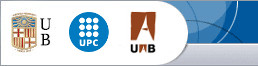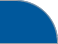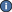Seminari de Geometria Algebraica de BarcelonaUB UPC UABIndex anual Seminari 2020/2021 Seminari 2019/2020 Seminari 2018/2019 Seminari 2017/2018 Seminari 2016/2017 Seminari 2015/2016 Seminari 2014/2015 Seminari 2013/2014 Seminari 2012/2013 Seminari 2011/2012 Seminari 2010/2011 Seminari 2009/2010 Seminari 2008/2009 Seminari 2007/2008 Seminari 2006/2007 Seminari 2005/2006 Seminari 2004/2005 Seminari 2003/2004 Seminari 2002/2003 Seminari 2001/2002Contacta amb els organitzadors: Josep Àlvarez Montaner Martí Lahoz Simone MarchesiSeminari de Geometria Algebraica 2020/2021Conferenciant Títol Data i hora Luis Narváez Universidad de Sevilla Contact: josep.alvarez at upc.es Differentially admissible algebras on a field of positive characteristic In the case of a base field of characteristic zero, Núñez-Betancourt (2013) introduced a new class of algebras on such a field, which later became called "differentially admissible algebras", which generalized a class of algebras that Mebkhout and the author had studied in 1991 as a general framework where there is a good theory of Bernstein-Sato polynomials and holonomous D-modules. In this session, we will propose a notion of "differentially admissible algebras" on a positive characteristic field and show some of the properties they share. Unfortunately, and as is well known, the theory of D-modules on a field of positive characteristic is more complicated and "uncertain" than on characteristic zero, although recently some generalizations of the "classical theory" have been introduced (holonomy, Bernstein-Sato polynomials, etc.) that are still being explored and that could provide interesting advances. In principle, differentially admissible algebras on a field of positive characteristic would add to this area to be explored. Divendres 1 d'octubre, 15h00, Aula T2, FMI-UB. Roberto Gualdi Universität Regensburg Contact: sombra at ub.edu On arithmetic divisors in Arakelov geometry Arakelov geometry offers an enjoyable framework to develop an arithmetic counterpart of the usual intersection theory. For varieties defined over the ring of integers of a number field, and inspired by the geometric case, one can define a suitable notion of arithmetic Chow groups and of an arithmetic intersection product. In a joint work with Paolo Dolce (Università degli Studi di Udine), we prove an arithmetic analogue of the classical Shioda-Tate formula, relating the dimension of the first Arakelov-Chow vector space of an arithmetic variety to some of its geometric invariants. In doing so, we also characterize numerically trivial arithmetic divisors, confirming part of a conjecture by Gillet and Soulé. Divendres 8 d'octubre, 15h00, Aula T2, FMI-UB. Rick Miranda Colorado State University, EEUU Contact: jroe at mat.uab.cat $$(-1)$$-curves in $$\mathbb P^r$$ We'll review some ways to generalize $$(-1)$$-curves to higher dimensions, and also review the Coxeter Group theory that applies to standard Cremona transformations based at points, and how the Weyl group is represented in the Chow ring. The goal is to better understand criteria for when a general $$(-1)$$-curve is a Cremona image of a line, and to apply these ideas to the Mori Dream Space cases of $$\mathbb P^r$$ blown up at points. Divendres 22 d'octubre, 15h00, Aula T2, FMI-UB. Sessió doble Ciro Ciliberto Università di Roma Tor Vergata, Itàlia Contact: jroe at mat.uab.cat Extensions of canonical curves and double covers A variety of dimension $$n$$ is said to be extendable $$r$$ times if it is the space section of a variety of dimension $$n+r$$ which is not a cone. I will recall some general facts about extendability, with special regard for extensions of canonical curves to $$K3$$ surfaces and Fano 3-folds. Then I will focus on double covers and on their extendability properties. In particular I will consider $$K3$$ surfaces of genus 2, that are double covers of the plane branched over a general sextic. A first result is that the general curve in the linear system pull back of plane curves of degree $$k\geq 7$$ lies on a unique $$K3$$ surface, so it is only once extendable. A second result is that, by contrast, if $$k\leq 6$$ the general such curve is extendable to a higher dimensional variety. In fact in the cases $$k=4,5,6$$, this gives the existence of singular index $$k$$ Fano varieties of dimensions 8, 5, 3, genera 17, 26, 37, and indices 6, 3, 1 respectively. For $k = 6$ one recovers the Fano variety $$\mathbb P(3, 1, 1, 1)$$, one of two Fano threefolds with canonical Gorenstein singularities with the maximal genus 37, found by Prokhorov. A further result is that this latter variety is no further extendable. For $$k=4$$ and $$5$$ these Fano varieties have been identified by Totaro. Divendres 22 d'octubre, 16h00, Aula T2, FMI-UB. Sessió doble Organitzada per la RGAS Jornada de Jóvenes Doctores en Geometría Algebraica 4-5 novembre, FMI-UB. Pablo González Mazón INRIA, Nice, França Contact: cdandrea at ub.edu Trilinear birational maps A trilinear rational map $$\phi: (\mathbb{P}_\mathbb{C}^1)^3 \dashrightarrow \mathbb{P}_\mathbb{C}^3$$ is a rational map whose entries are trilinear polynomials. If it admits an inverse rational map $$\phi^{-1}: \mathbb{P}_\mathbb{C}^3 \dashrightarrow (\mathbb{P}_\mathbb{C}^1)^3$$, we say that $$\phi$$ is a trilinear birational map. Similarly, a bilinear birational map is a birational map $$\psi: (\mathbb{P}_\mathbb{C}^1)^2 \dashrightarrow \mathbb{P}_\mathbb{C}^2$$ with bilinear entries. Bilinear birational maps can be efficiently used in Computer Aided Design, and are already understood well. More explicitly, the set of bilinear birational maps is an irreducible locally closed hypersurface in $$\mathbb{P}^{11}$$. Moreover, the base scheme of $$\psi$$ is always a closed point, and the minimal bi-graded free resolution of its base ideal is Hilbert-Burch, with two linear syzygies. In our work, we answer the analogous questions about the algebraic structure, geometry of the base scheme, and resolution of the base ideal, for the 3-dimensional counterpart. Namely, we give the set (of classes) of $$\text{Bir}_{(1,1,1)}$$ of trilinear birational maps the structure of an algebraic set, and describe its irreducible components. Secondly, we provide the complete list of possible base schemes for $$\phi$$, up to isomorphism between these. Finally, we classify all the possible tri-graded minimal free resolutions of the base ideal of $$\phi$$. Divendres 12 de novembre, 15h00, Aula T2, FMI-UB. Francesc Fité UB Contact: On a local-global principle for quadratic twists of abelian varieties Let $$A$$ and $$A'$$ be abelian varieties defined over a number field $$k$$. In the talk I will consider the following question: Is it true that $$A$$ and $$A'$$ are quadratic twists of one another if and only if they are quadratic twists modulo $$p$$ for almost every prime $$p$$ of $$k$$? Serre and Ramakrishnan have given a positive answer in the case of elliptic curves and a result of Rajan implies the validity of the principle when $$A$$ and $$A'$$ have trivial geometric endomorphism ring. Without restrictions on the endomorphism ring, I will show that the answer is affirmative up to dimension $$3$$, but that it becomes negative in dimension $$4$$. The proof builds on Rajan's result and uses a Tate module tensor decomposition of an abelian variety geometrically isotypic (the latter obtained in collaboration with Xavier Guitart). Divendres 17 de desembre, 15h00, Aula T2, FMI-UB.Universitat de Barcelona Universitat Politècnica de Catalunya Universitat Autònoma de Barcelona